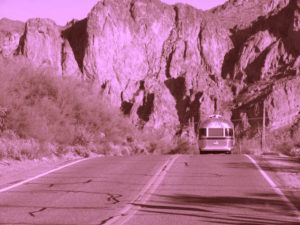## Put it TogetherAt the beginning of the module, Joan had applied for a credit card with air miles so she could go on a trip. To earn enough miles for the airfare, Joan has planned to use her air miles card for the following things each month:

• gas = $30 • car insurance =$40
• mobile phone bill = $65 • groceries =$150
• entertainment – restaurants, movies, and live music = $50 The total monthly amount Joan plans to spend on bills is$335.

Each dollar she spends gives her 1.25 air miles, and she expects to need about 12,000 air miles to buy the ticket she wants. The credit card company started Joan off with 100 air miles just for signing up.

Let’s help Joan plan for her trip by figuring out how much she should put on her card until she has enough air miles. Because the amount of miles earned depends on the number of dollars spent this is a good candidate as a function. The function will be linear  because the number of miles earned per dollar spent is constant.

Since we are trying to figure out how long she needs to save to get to a certain number of air miles, we will make dollars spent the input variable in our function. The output will be the number of air miles earned.

$A(d)=\text{Number of air miles earned as a function of dollars spent}$

Joan has earned 100 air miles for signing up, so we need to add that as a constant to the function.

$A(d)=100+1.25(d)$

If we put in $335, we will see how many miles she earns in the first month. $A(335)=100+1.25(335)=100+418.75=518.75$ So after the first month, Joan has 518 air miles. Thats’ not quite 12,000. We could keep putting in multiples of 335 to see how much she has to spend before she has enough miles, or we could just solve for it: $\begin{array}12,000=100+1.25(d)\\=11,900=1.25(d)\\=9520=d\end{array}$ This means she will need to spend$9520 to have 12,000 air miles. If she spends \$335 per month for sure, then $\frac{9520}{335}$ will give the number of months until she has enough miles to buy her ticket.

$\frac{9520}{335}=28.42$

Joan will need just over two years to have enough miles, which is perfect because that’s how long it will take for her to finish her degree.  She can celebrate graduating with a trip she earned with free air miles. Now Joan just needs to figure out where she wants to go. . .